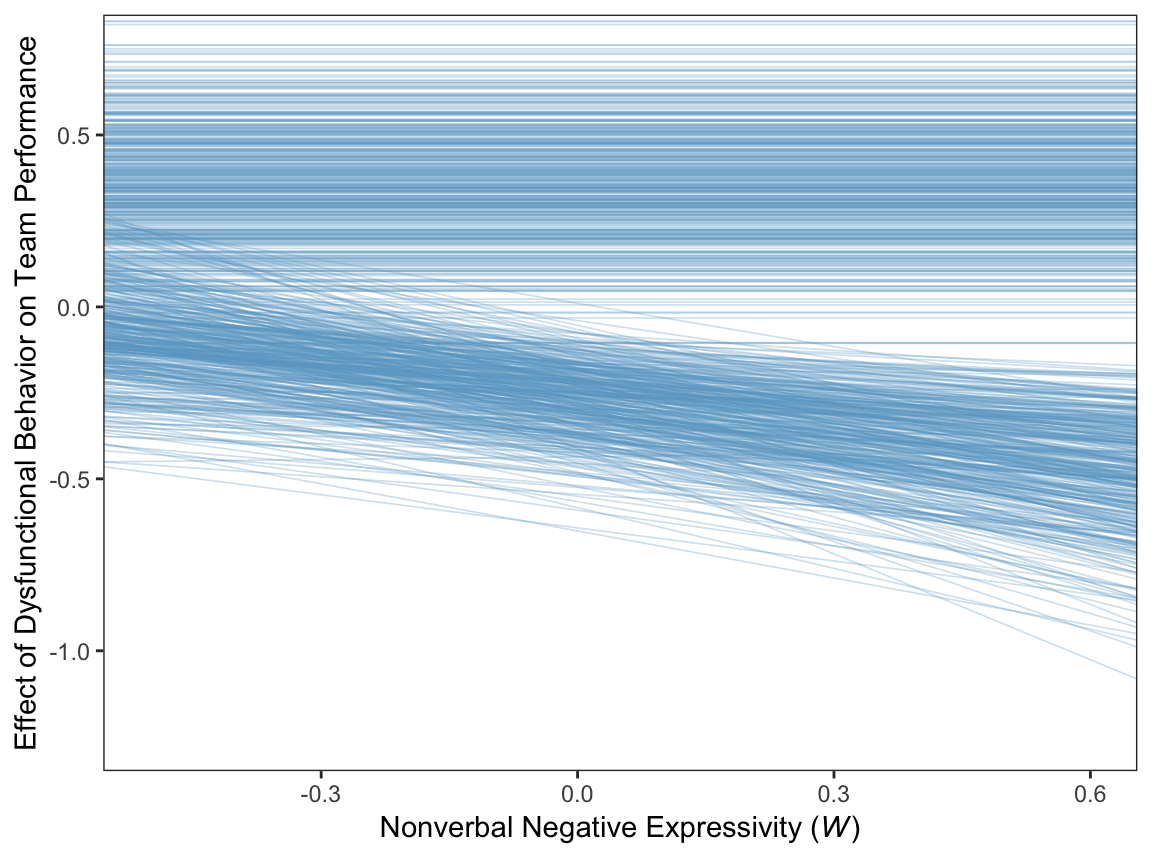## 11.5 Quantifying and visualizing (conditional) indirect and direct effects.

#### 11.5.0.1 The conditional indirect effect of $$X$$.

Here’s how to get the posterior summaries corresponding to the last two columns in Table 11.2.

post <-
posterior_samples(model1) %>%
mutate(Conditional effect of M when W is -0.531 = b_perform_negtone + b_perform_negtone:negexp*-0.531,
Conditional effect of M when W is -0.006 = b_perform_negtone + b_perform_negtone:negexp*-0.060,
Conditional effect of M when W is 0.600 = b_perform_negtone + b_perform_negtone:negexp*0.600,

Conditional indirect effect when W is -0.531 = b_negtone_dysfunc*(b_perform_negtone + b_perform_negtone:negexp*-0.531),
Conditional indirect effect when W is -0.006 = b_negtone_dysfunc*(b_perform_negtone + b_perform_negtone:negexp*-0.060),
Conditional indirect effect when W is 0.600 = b_negtone_dysfunc*(b_perform_negtone + b_perform_negtone:negexp*0.600))

post %>%
select(starts_with("Conditional")) %>%
gather() %>%
mutate(key = factor(key, levels = c("Conditional effect of M when W is -0.531",
"Conditional effect of M when W is -0.006",
"Conditional effect of M when W is 0.600",

"Conditional indirect effect when W is -0.531",
"Conditional indirect effect when W is -0.006",
"Conditional indirect effect when W is 0.600"))) %>%
group_by(key) %>%
summarize(mean = mean(value),
sd = sd(value),
ll = quantile(value, probs = .025),
ul = quantile(value, probs = .975)) %>%
mutate_if(is.double, round, digits = 3)
## # A tibble: 6 x 5
##   key                                            mean    sd     ll     ul
##   <fct>                                         <dbl> <dbl>  <dbl>  <dbl>
## 1 Conditional effect of M when W is -0.531     -0.168 0.212 -0.573  0.26
## 2 Conditional effect of M when W is -0.006     -0.409 0.139 -0.687 -0.14
## 3 Conditional effect of M when W is 0.600      -0.747 0.169 -1.08  -0.429
## 4 Conditional indirect effect when W is -0.531 -0.104 0.141 -0.407  0.165
## 5 Conditional indirect effect when W is -0.006 -0.254 0.114 -0.51  -0.066
## 6 Conditional indirect effect when W is 0.600  -0.464 0.167 -0.824 -0.177

#### 11.5.0.2 The direct effect.

The direct effect for his model is b_perform_dysfunc in brms. Here’s how to get its summary values from posterior_summary().

posterior_summary(model1)["b_perform_dysfunc", ] %>% round(digits = 3)
##  Estimate Est.Error      Q2.5     Q97.5
##     0.369     0.184     0.006     0.730

### 11.5.1 Visualizing the direct and indirect effects.

For Figure 11.7 we’ll use the first 400 HMC iterations.

post <-
post %>%
mutate(-0.7 = b_negtone_dysfunc*(b_perform_negtone + b_perform_negtone:negexp*-0.7),
0.7 = b_negtone_dysfunc*(b_perform_negtone + b_perform_negtone:negexp*0.7))

post %>%
select(b_perform_dysfunc, -0.7:0.7) %>%
gather(key, value, -b_perform_dysfunc) %>%
mutate(negexp = key %>% as.double(),
iter = rep(1:4000, times = 2)) %>%
filter(iter < 401) %>%

ggplot(aes(x = negexp, group = iter)) +
geom_hline(aes(yintercept = b_perform_dysfunc),
color = "skyblue3",
size = .3, alpha = .3) +
geom_line(aes(y = value),
color = "skyblue3",
size = .3, alpha = .3) +
coord_cartesian(xlim = c(-.5, .6),
ylim = c(-1.25, .75)) +
labs(x = expression(paste("Nonverbal Negative Expressivity (", italic(W), ")")),
y = "Effect of Dysfunctional Behavior on Team Performance") +
theme_bw() +
theme(panel.grid = element_blank())Since the b_perform_dysfunc values are constant across $$W$$, the individual HMC iterations end up perfectly parallel in the spaghetti plot. This is an example of a visualization I’d avoid making with a spaghetti plot for a professional presentation. But hopefully it has some pedagogical value, here.